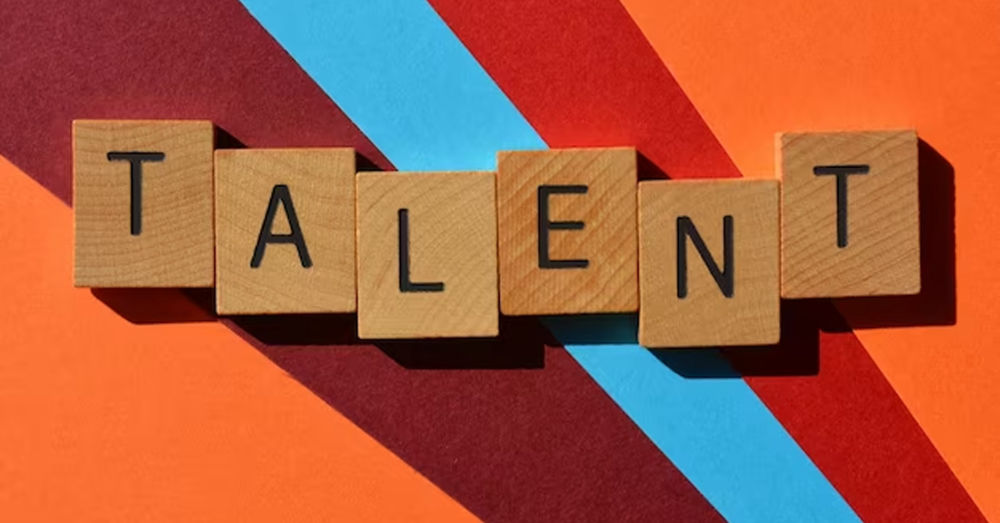• Save

# Math Aptitude Questions and AnswersLooking for help with math questions for competitive exams? Look no further! Our comprehensive guide includes general math questions and answers to help you prepare for your next exam. Whether you're studying for the SAT, GRE, GMAT, or any other exam, we've got you covered. Our expert team has put together a list of commonly asked math questions and provides clear easy-to-understand explanations. From algebra to geometry, trigonometry to calculus, we cover all the essential topics you'll need to know to ace your math exam. So why wait? Start preparing now and boost your confidence for your next test!

## Math Aptitude Questions and Answers

Here, I am sharing Math Aptitude Questions and Answers related to Quaditral Equations, coordinate Geometry, Measurement, Equations, Statics and Data Analysis for upcoming competitive exams like SSC, RRB, Bank and other government exams. These Math Aptitude Questions and Answers will be very helpful for you to crack any exams.

Also, Read Latest Current Affairs Questions 2022: Current Affairs Today

Students can quickly get free General Knowledge Mock Test and Current Affairs Mock Test on this platform for online exam practice to obtain good marks in competitive exams.

## Information Technology Questions for REET Level 2

Q :

What should come in place of the question mark (?) in the following question?
24% of 420 × 50% of 74 = ?

(A) 3729.6

(B) 101.17

(C) 68.432

(D) 970.46

(E) None of these

Q :

A semi-circular sheet of metal of diameter 28 cm. is bent into an open conical cup. The depth of the cup is approximately

(A) 15 cm.

(B) 14 cm.

(C) 11 cm.

(D) 12 cm.

Q :

The lateral surface area of a cylinder is 792cm2. If its height is 14cm, then its volume (in cm3) is? [Take π = 22/7 ]

(A) 2564

(B) 3929

(C) 1243

(D) 3564

Q :

The side of a square is 8 cm. If a square is made by joining the mid points of each sides of this square and this process goes up to infinity. Calculate the sum of areas of all the squares made.

(A) 128 $$cm^2$$

(B) 256 $$cm^2$$

(C) 64 $$cm^2$$

(D) 32 $$cm^2$$

Q :

A copper wire is bent in the form of an equilateral triangle and has area 121√3 cm2 . If the same wire is bent into the form of a circle, the area (in cm2) enclosed by the wire is (Take pi= 22 /7)

(A) 322.5$$cm^2$$

(B) 286.5 $$cm^2$$

(C) 388.5 $$cm^2$$

(D) 346.5$$cm^2$$

Q :

The length, breadth and height of a hall are 144 m, 180 m and 384 m respectively. The length of the longest scale that can measure the three dimensions of the hall exactly is:

(A) 30 m

(B) 12 m

(C) 15 m

(D) 25 m

Q :

A water tank is 15 meter long. 10 meter wide and 3 meter deep. The total cost to repair its four walls and bottom at the rate of 24 rupees per square meter is?

(A) Rs. 9600

(B) Rs. 4800

(C) Rs. 3600

(D) Rs.7200

Q :

The diameters of two cones are equal. If their slant heights are in the ratio 5: 2, then the ratio of curved surface areas of the cones is _________ .

(A) 2 : 5

(B) 5 : 2

(C) 25 : 4

(D) 4 : 5

Q :

If the diagonals of a rhombus are 24cm and 10cm, then the perimeter of the rhombus is ___________.

(A) 48cm

(B) 52cm

(C) 40cm

(D) 56cm

Q :

A rectangular box measures internally 1.6 m long, 1 m broad and 60 cm deep. The number of cubical block each of edge 20 cm that can be packed inside the box is:

(A) 60

(B) 53

(C) 30

(D) 120